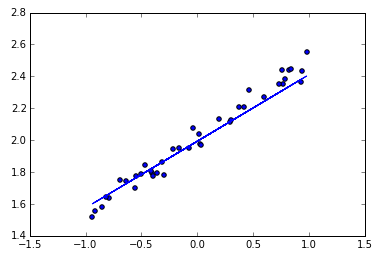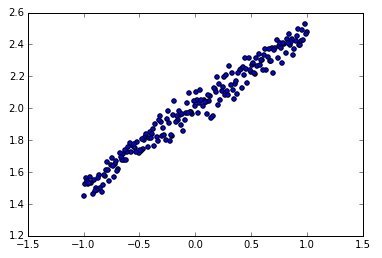# Regressor 回归## 导入模块并创建数据 ¶

models.Sequential，用来一层一层一层的去建立神经层； layers.Dense 意思是这个神经层是全连接层。

import numpy as np
np.random.seed(1337)  # for reproducibility
from keras.models import Sequential
from keras.layers import Dense
import matplotlib.pyplot as plt # 可视化模块

# create some data
X = np.linspace(-1, 1, 200)
np.random.shuffle(X)    # randomize the data
Y = 0.5 * X + 2 + np.random.normal(0, 0.05, (200, ))
# plot data
plt.scatter(X, Y)
plt.show()

X_train, Y_train = X[:160], Y[:160]     # train 前 160 data points
X_test, Y_test = X[160:], Y[160:]       # test 后 40 data points## 建立模型 ¶

model = Sequential()


## 激活模型 ¶

# choose loss function and optimizing method
model.compile(loss='mse', optimizer='sgd')


## 训练模型 ¶

# training
print('Training -----------')
for step in range(301):
cost = model.train_on_batch(X_train, Y_train)
if step % 100 == 0:
print('train cost: ', cost)

"""
Training -----------
train cost:  4.111329555511475
train cost:  0.08777070790529251
train cost:  0.007415373809635639
train cost:  0.003544030711054802
"""


## 检验模型 ¶

# test
print('\nTesting ------------')
cost = model.evaluate(X_test, Y_test, batch_size=40)
print('test cost:', cost)
W, b = model.layers.get_weights()
print('Weights=', W, '\nbiases=', b)

"""
Testing ------------
40/40 [==============================] - 0s
test cost: 0.004269329831
Weights= [[ 0.54246825]]
biases= [ 2.00056005]
"""


## 可视化结果 ¶

# plotting the prediction
Y_pred = model.predict(X_test)
plt.scatter(X_test, Y_test)
plt.plot(X_test, Y_pred)
plt.show()